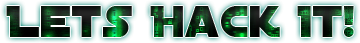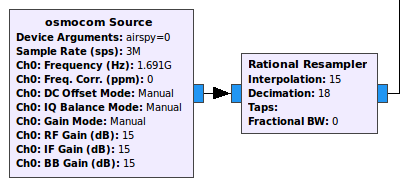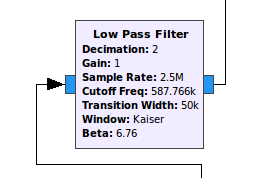# Decimating and filtering to desired sample rate

The next step is to decimate to reach the 2.5e6 of sample rate. For the airspy mini that is 15/18 of the 3e6 sample rate. So lets create a Rational Resampler block and put 15 as interpolation and 18 as decimation. The taps can be empty since it will auto-generate. This is not very optimal, but will work for now. I will release a better version for each SDR in the future.Now we have 2.5 Msps and we need to decimate by two. But we will also lowpass the input to something close our rate. So let’s create a Low Pass filter with Decimation as 2Sample Rate as 2.5e6Cut Off Frequency as symbol_rate * 2 (that is 587766)Transition Width as 50e3.After that we will have the sample rate is 1.25e6